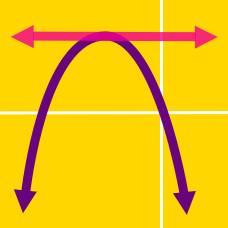Algebra

# Completing the Square - Problem Solving

Consider two functions \begin{align} f(x) &= -4x^2 + 14 x + 4 \\ g(x) &= -7 x^2 + 8 x + 3. \end{align} Let $$a$$ be the maximum value of $$f(x) + g(x) ,$$ and $$b$$ the value of $$x$$ at that moment. Then what is the value of $$a+b?$$

For real numbers $$x$$ and $$y$$, the polynomial $x^2+2y^2+2xy-8x-10y+30$ has a minimum value $$m$$ at $$x=a$$ and $$y=b$$. What is the value of $$abm$$?

Let $$f(x)= 5 x + 60$$ and $$g(x)= 4 x + 80.$$ If $$a$$ is the minimum value of $$f(x) \times g(x)$$ and $$b$$ is the value of $$x$$ at that moment, what is the value of $$b-a?$$

Consider two points $$A=(2,5)$$ and $$B=(4,4)$$, and a third point $$P=(a, 0)$$ on the $$x$$-axis. If the minimum value of $$\overline{PA}^2+\overline{PB}^2$$ is $$c$$, what is $$a+c$$?

Consider the parabola $y = x^2 + k^4 + p^4 -2k^2 x -2p^2x + 2k^2p^2 + 36,$ where $$k$$ and $$p$$ are parameters. If the vertex of the parabola lies on the line $y = \frac{ 1 }{25} x,$ what is the value of $$k^2 + p^2?$$

×